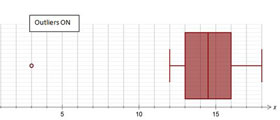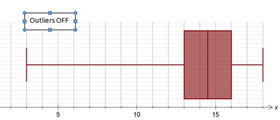# Identifying outliers

In box plots, an outlier can be identified when using technology. This function may be turned on or off.

In the Australian Curriculum: Mathematics, an outlier is described as any data point that is more than 1.5 times the interquartile range (IQR, where IQR = Q3 – Q1) from either the upper quartile (Q3) or the lower quartile (Q1).Produced with outlier function 'on'.Produced with outlier function 'off'.

In the box plot above, any value less than 8.5 is an outlier.

Q1 – (1.5 $$\times$$ IQR)= 13 – (1.5 $$\times$$ 3) = 8.5

The Beware of Outliers: Student Worksheet provides an activity based on outliers and the mean. You can also download the Beware of Outliers: Teacher Notes.